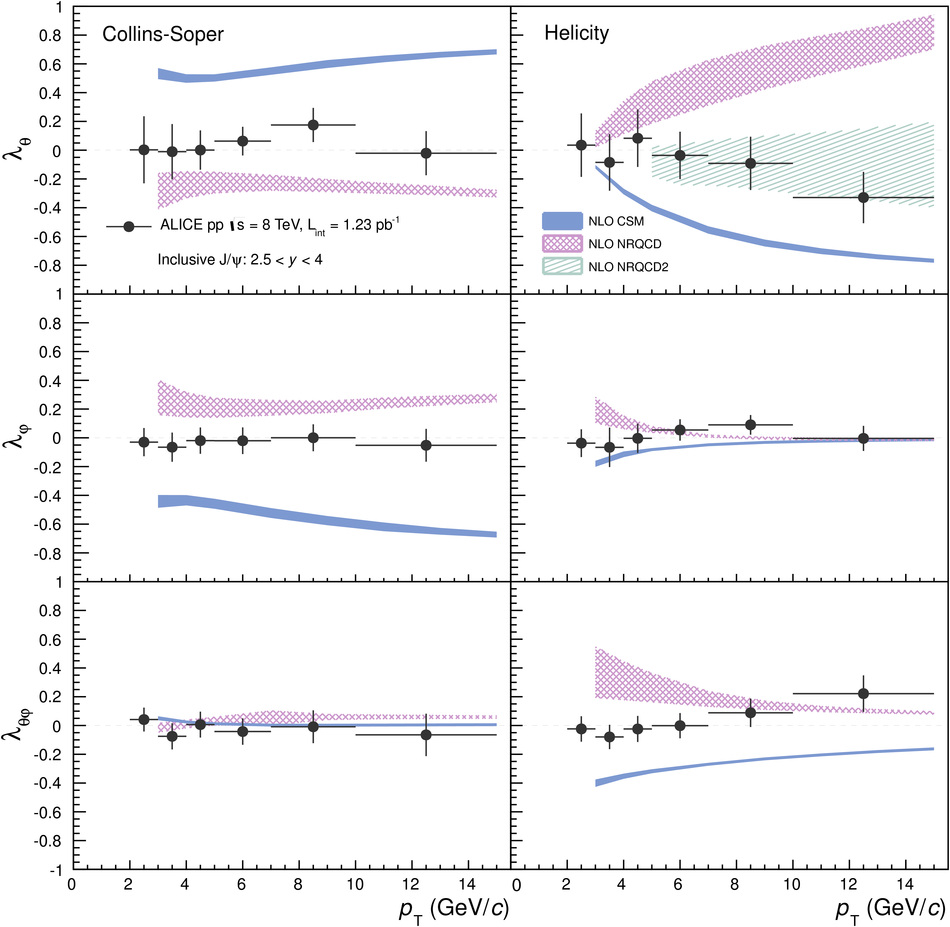# Figure 4

 Inclusive J/$\psi$ polarization parameters in pp collisions at $\sqrt{s} = 8$~TeV (black points, error bars represent the total uncertainties) compared with model predictions: NLO CSM~ (blue filled bands), NRQCD~ (red shaded bands) and NRQCD2~ (light blue hatched band). Left and right plots show the results in the Collins-Soper and helicity frames, respectively, for $\lambda_\theta$ (top plots), $\lambda_\varphi$ (middle plots) and $\lambda_{\theta\varphi}$ (bottom plots).